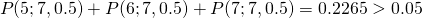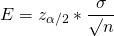# Statistics for Machine Learning

### Let's take a look at what we encounter when studying Machine Learning: statistics. Explore different types of statistics, such as inferential statistics and statistical test.

· AI Zone · Analysis
Save
3.81K Views

one of the first things that one encounters when studying machine learning is a barrage of terms like inferential statistics, statistical test, statistical hypothesis, null hypothesis, alternative hypothesis, p-value, probability distribution...the list goes on and on.

this may prove discouraging for those who are not familiar with statistics. the present post aims to provide a gentle introduction to all those terms and concepts.

## inferential statistics

the goal of inferential statistics is to examine the relationships between variables within a sample and then make predictions about how those variables relate to a larger population.

### inference in natural sciences

this way of working — analyzing a sample and then make generalizations/predictions for a larger population — is not exclusive of statistics. on the contrary, this is the way natural sciences work.

for instance, newton's theory of gravitation combines the masses of objects interacting and the distance between them according to the well-known formula:the validity of the model is established by comparing the results predicted by the formula with the empirical results.

### inference in statistics

in statistics, there are no formulas combining variables through some kind of rule. instead, the variables are assumed to take random values .

this is another way to say that we do not know the laws that govern that phenomenon. or maybe we do, but the system is so complex and with so many variables, that traditional computations are not feasible.

for example, the field of physics called statistical mechanics studies physical systems with a large degree of freedom. while classical mechanics can deal with problems involving a few bodies, see n-body problem , statistical mechanics is used for the study of systems comprising billions of particles.

### statistical inference methods

even though the variables of a statistical model are random, there must be some way to predict their behavior, otherwise, it would be impossible to test the model.

normally, inferential statistics operate in two ways:

• confidence intervals : by analyzing a sample, the value of a parameter of an entire population is expressed in terms of an interval and the degree of confidence that the parameter is within the interval. this way of working results in statements like "there is a 95% probability that the mean height of all american men has a value in the interval [165 cm, 175 cm]"
• hypothesis testing: by analyzing a sample, a claim about the population is made in terms of a level of significance. as a consequence, it's possible to make statements like: "a dice is biased with a 95% level of confidence (the null hypothesis was rejected at a 5% level of significance)"

the following sections show some examples of each method to better understand how they work.

## hypothesis testing

statistical models represent the probability of their random variables taking a certain value. these representations are called probability distributions.

there are discrete probability distributions (when the variables can only take values from a finite set) and continuous probability distributions (when there are infinite values).

for instance, the result of tossing a coin is a random variable. a model to represent this phenomenon is the discrete uniform distribution , where each of the 2 possible results have the same probability (1/2). we say that a coin is fair if the results after tossing the coin a sufficiently high number of times are in accordance with the model.

let's suppose that we toss a coin 7 times and get 5 heads. is this a fair coin? to answer this question, we will prove that the probability of getting this result according to our model is very low and, as a result, it is unlikely to be a fair coin. how low? by convention, the threshold is set to a value of 0.05.

the above paragraph can be expressed in statistics jargon:

we will reject the null hypothesis, (that states that the coin is a fair one), by showing that the p-value of getting at least 5 heads out of 7 tosses according to the null hypothesis is statistically significant (less than the significance level threshold of 0.05) and, as a consequence, the alternative hypothesis, (that states that the coin is not fair), will be favored.

in order to calculate the p-value (the probability of getting at least 5 heads out of 7 tosses), we can use the binomial distribution.

a binomial distribution with parameters n and p determines the probability of getting a certain number of successes in a sequence of n independent experiments whose outcome is a random variable taking the value true (with probability p) or false (with probability 1-p)in our case, we want to calculate the probability of getting at least 5 heads in a sequence of 7 experiments and, because we are under the null hypothesis that states that the coin is fair, the probability of getting a head in each individual experiment is 0.5. with all this information, the p-value will betherefore, the result is not statistically significant, meaning that is not conclusive enough to reject the null hypothesis.

## confidence intervals

a confidence interval is a numerical range, which, most likely, contains the actual population value. the probability that the interval contains the population value is called the confidence level.

see https://www.thoughtco.com/calculating-a-confidence-interval-for-a-mean-3126400 for more details.

let's suppose that we want to determine the mean height of a population of men. we will first measure the mean height of a sample of 15 men and then build a confidence interval to extrapolate the results to the whole population.

after measuring the height of the individuals in the sample, the following results are obtained:

``data = [169.94,173.12,166.3,162.79,174.34,185.83,169.63,173.41,172.92,165.0,169.29,160.28,168.38,171.87,163.66]``

the mean and standard deviation corresponding to these results are 169.78 and 6.16 respectively.

the margin of error is given by the formula:that combines the critical value, the standard deviation and the sample size.

the critical value for 14 (size of the sample - 1) degrees of freedom and 90% level of confidence is 1.34503 ( https://goodcalculators.com/student-t-value-calculator )

therefore e = 2.14 and the observed mean 169.78 falls in a 90% confidence interval from 167.64 to 171.92.

as a result, we can state that the true mean of the population falls in the interval (167.64, 171.92) with a 90% level of confidence.

note : to apply the margin of error formula, we are assuming that the data obtained from the sample follows a normal distribution.

## simulation

arguably, simulations run on computers are a more intuitive approach than analytical/theoretical methods. this section, taking the examples on https://docs.python.org/3/library/random.html as inspiration, shows how to solve the previous problems using simulation.

### hypothesis testing

let's try to replicate the result obtained when studying the fairness of a coin giving 5 heads out of 7 tosses.

in order to do that, we will run thousands of trials, each trial consisting of tossing the coin 7 times and counting the number of heads. the resulting value of each toss is taken from the discrete uniform distribution, that is the model that describes the coin behavior.

the p-value will be calculated as the fraction of trials in which the number of heads is at least 5.

``````from random import choices
n = 7 #number of tosses
minnumbersuccesses = 5 #number of heads
p = 0.5 #probability of getting a head on a single experiment
trials = 100000 #number of trials
sum(trial() for i in range(trials))/trials``````

after running the simulation, the result is 0.2261, that is very close to the theoretical value 0.2265.

### confidence intervals

to replicate the results of the confidence interval, we will use a technique called bootstrapping consisting in resampling with replacement.

based on the description of the method on https://en.wikipedia.org/wiki/bootstrapping_(statistics)#approach , we take samples (resamples) from the original sample. each resample has the same number of elements as the original sample and, possibly, repeat elements.

``````from random import choices
def bootstrap(data):
return choices(data, k=len(data))

n = 10000
means = sorted([mean(bootstrap(data)) for i in range(n)])
print(f'resample mean: {mean(means):.2f}')
#90% confidence interval goes from 5% to 95%
print(f'lower bound: {means[round(n*.05)]:.2f}')
print(f'upper bound: {means[round(n*.95)]:.2f}')``````

the result of running the program is:

``````resample mean: 169.80
lower bound: 167.37
upper bound: 172.43``````

the mean of the resamples is 169.80, very similar to the value 169.78 obtained for the original sample.

according to the above results, the true mean of the population falls in the interval (167.37, 172.43) with a 90% level of confidence. this value is very similar to the interval (167.64, 171.92) calculated analytically.

one of the advantages of this technique is that it does not make any assumption about the distribution followed by the sample data (as opposed to the analytical method, that expected the data to follow the normal distribution)

### coin problem revisited

when implementing the coin simulation, we had a model (the discrete uniform distribution) to base our simulation on.

however, in many real-life situations there is no such a model; for instance, when a drug is tested by comparing to a control group (placebo).

to illustrate this idea, let's assume that the underlying model that describes the coin problem is unknown. how can the fairness of the coin be determined then?

instead of the uniform distribution model, we need some other reference. that reference will be another coin, a "magic" coin that we take as our gold standard of coin fairness. any other coin will be considered fair as long as its results are in accordance with the reference coin.

getting back to the problem, let's imagine that the 5 heads were obtained in this sequence:

``coin = [1, 1, 1, 1, 1, 0, 0]``

then, we toss our magic coin 7 times and get this sequence:

``magic_coin = [1, 1, 0, 0, 0, 1, 0]``

to solve this problem we will use resampling in the form of permutation tests (before we dealt with resampling in the form of bootstrapping).

under the null hypothesis, the results obtained for each coin are indistinguishable. because of that, it would be possible to interchange the results of both coins without noticing any difference.

to run our hypothesis test, we will shuffle the original results thousands of times and count how many times the difference of the means of the coins is more extreme than the original difference. that will be the p-value.

``````from statistics import mean
from random import shuffle

coin = [1,1,1,1,1,0,0]
magic_coin = [1,1,0,0,0,1,0]
observed_diff = mean(coin) - mean(magic_coin)

n = 10000
count = 0
combined = coin + magic_coin
for i in range(n):
shuffle(combined)
new_diff = mean(combined[:len(coin)]) - mean(combined[len(coin):])
count += (new_diff >= observed_diff)

print(f'{n} label reshufflings produced {count} instances with a difference')
print(f'at least as extreme as the observed difference of {observed_diff:.2f}.')
print(f'the p-value is {count / n:.4f}')
``````

and the result of running this simulation is:

``````10000 label reshufflings produced 2928 instances with a difference
at least as extreme as the observed difference of 0.29.
the p-value is 0.2928``````

as 0.2928 > 0.05, the null hypothesis cannot be rejected and therefore, there is no reason to suspect that our coin is not a fair one.

Topics:
artificial intelligence, machine learning, statistics, hypothesis testing, confidence intervals

Published at DZone with permission of Francisco Alvarez, DZone MVB.

Opinions expressed by DZone contributors are their own.